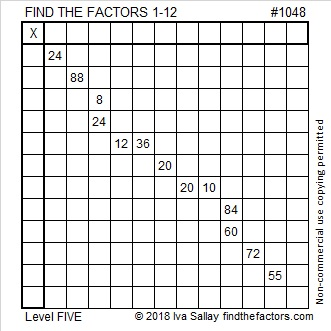# 1048 and Level 5

What is the common factor needed for 8 and 24 to make this puzzle work? Is it 2, 4, or 8? How about for 12 and 36? Is it 3, 4, 6, or 12? Don’t guess which common factor to use. In each case, all but one of the choices will be eliminated by using logic. It won’t be easy, but if you are determined, you can solve this puzzle.Print the puzzles or type the solution in this excel file: 12 factors 1044-1053

Here are some facts about the number 1048:

• 1048 is a composite number.
• Prime factorization: 1048 = 2 × 2 × 2 × 131, which can be written 1048 = 2³ × 131
• The exponents in the prime factorization are 1 and 3. Adding one to each and multiplying we get (3 + 1)(1 + 1) = 4 × 2 = 8. Therefore 1048 has exactly 8 factors.
• Factors of 1048: 1, 2, 4, 8, 131, 262, 524, 1048
• Factor pairs: 1048 = 1 × 1048, 2 × 524, 4 × 262, or 8 × 131
• Taking the factor pair with the largest square number factor, we get √1048 = (√4)(√262) = 2√262 ≈ 32.3728281048 can be written as the difference of two squares two different ways:
263² – 261² = 1048
133² – 129² = 1048

1048 can also be expressed as 2 times a factor pair 3 different ways:
2(524)(1)
2(262)(2)
2(131)(4)

Those facts make 1048 a leg in these FIVE obscure Pythagorean triples:
1048-137286-137290 calculated from 263² – 261², 2(263)(261), 263² + 261²
1048-34314-34330 calculated from 133² – 129², 2(133)(129), 133² + 129²
1048-274575-274577 calculated from 2(524)(1), 524² – 1², 524² + 1²
1048-68640-68648 calculated from 2(262)(2), 262² – 2², 262² + 2²
1048-17145-17177 calculated from 2(131)(4), 131² – 4², 131² + 4²

This site uses Akismet to reduce spam. Learn how your comment data is processed.